# ORELA Middle Grades Math: Properties of Functions Chapter Exam

Exam Instructions:

Choose your answers to the questions and click 'Next' to see the next set of questions. You can skip questions if you would like and come back to them later with the yellow "Go To First Skipped Question" button. When you have completed the practice exam, a green submit button will appear. Click it to see your results. Good luck!

### Page 1

#### Question 2 2. Use the graph to find f(f(2)).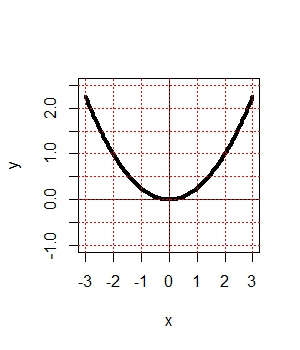#### Question 5 5. Remembering function notation, we want x to be w. Therefore, if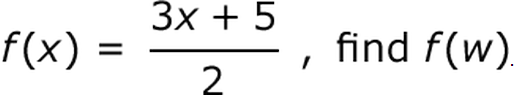### Page 3

#### Question 15 15. In the figure shown below, the graph of the green function is a translation of the graph of the red function. If the expression for the red function is g(x) = r(x), which of the following is the equation of the green function?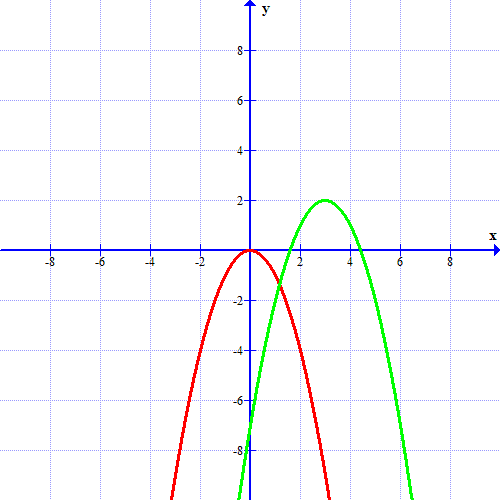### Page 4

#### Question 18 18. The revenue of a company is represented by R(x) and their costs are represented by C(x). What is the profit function R(x) - C(x)?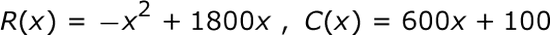### Page 5

#### Question 25 25. Using the graph, find f(f(-2)).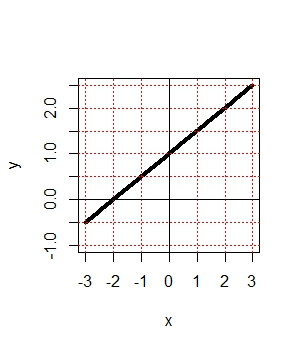### Page 6

#### Question 26 26. Find the domain of f(x)/g(x), if: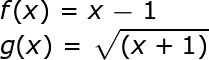#### Question 27 27. Evaluate.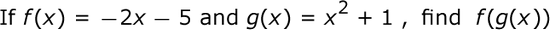#### ORELA Middle Grades Math: Properties of Functions Chapter Exam Instructions

Choose your answers to the questions and click 'Next' to see the next set of questions. You can skip questions if you would like and come back to them later with the yellow "Go To First Skipped Question" button. When you have completed the practice exam, a green submit button will appear. Click it to see your results. Good luck!

Support